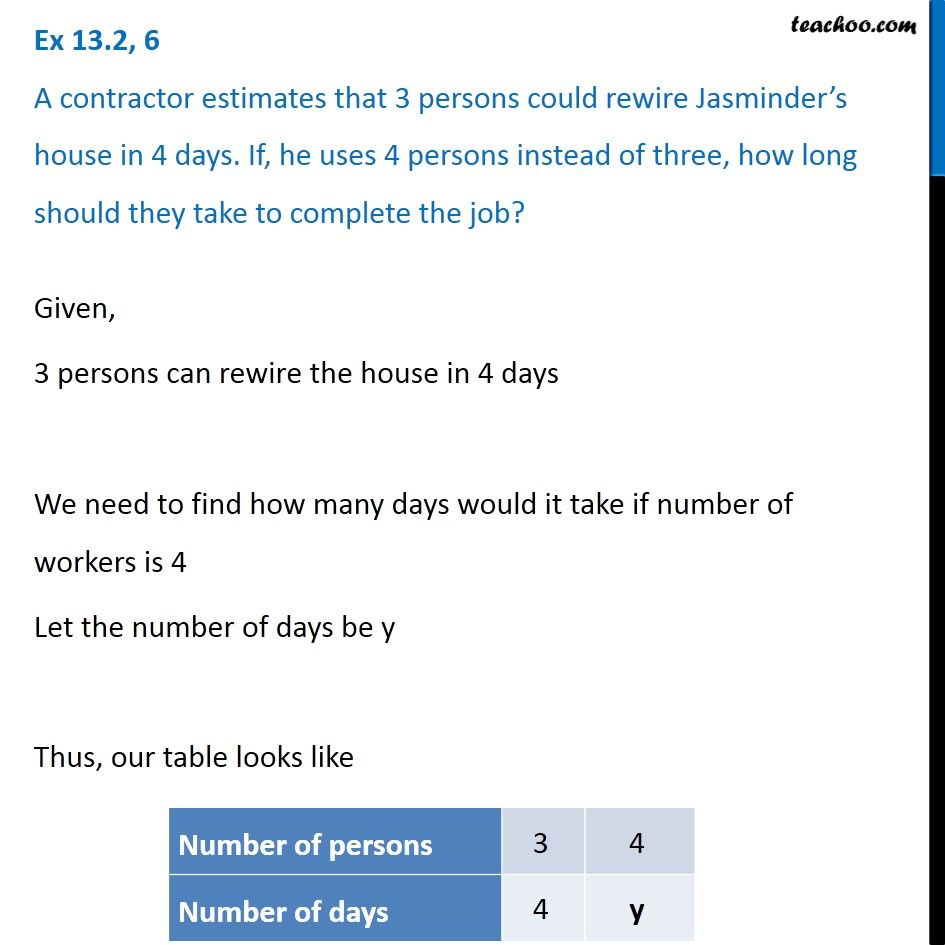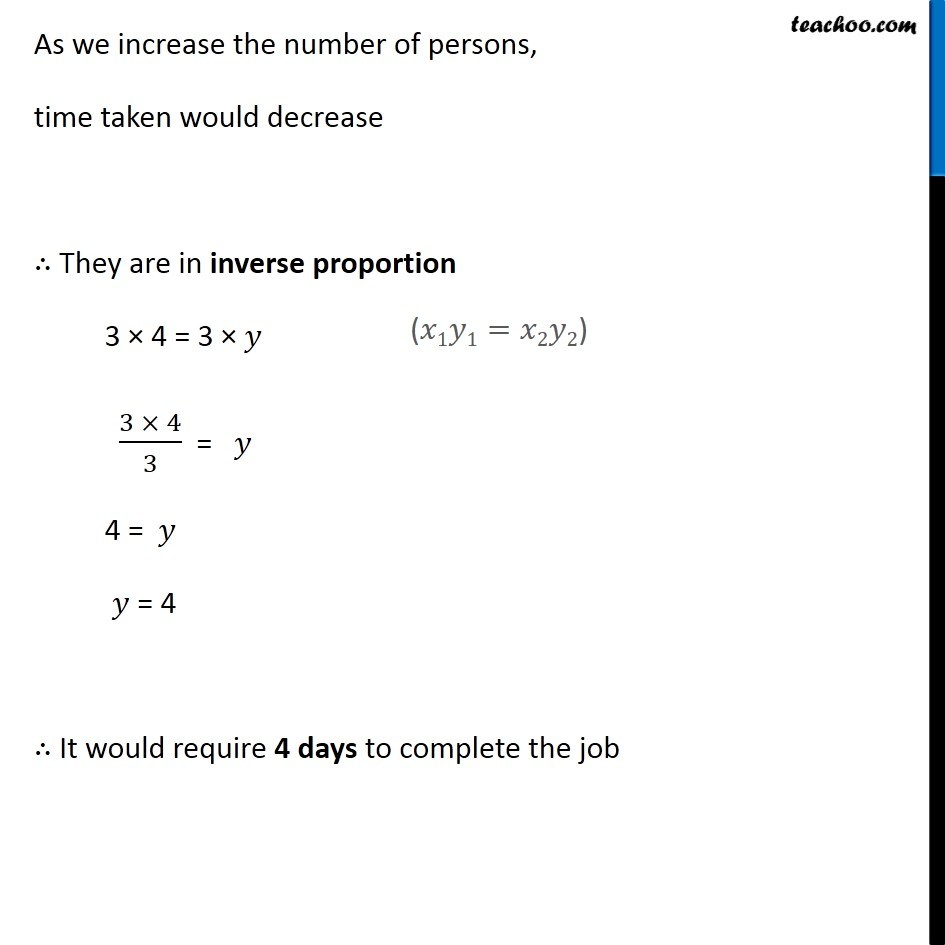Inverse Proportion

Chapter 13 Class 8 Direct and Inverse Proportions
Concept wise### Transcript

Ex 13.2, 6 A contractor estimates that 3 persons could rewire Jasminder’s house in 4 days. If, he uses 4 persons instead of three, how long should they take to complete the job? Given, 3 persons can rewire the house in 4 days We need to find how many days would it take if number of workers is 4 Let the number of days be y Thus, our table looks like As we increase the number of persons, time taken would decrease ∴ They are in inverse proportion 3 × 4 = 3 × 𝑦 (3 × 4)/3 = 𝑦 4 = 𝑦 𝑦 = 4 ∴ It would require 4 days to complete the job